The 3 most Used command in 3D in AutoCAD | 12CAD.com

# The 3 most Used command in 3D in AutoCAD

Are you a beginner? Do u have so many question about where to start drawing in 3D with AutoCAD? Have you mastered your 2D yet or do you just want to dive right into the “real world” the most interesting part which I name the 3D?

Here is from my opinion the most used command in 3D, and by far the 3 commands you should master on your first day on your adventure in the 3D world.

They are, UNION, SUBTRACT and INTERSECT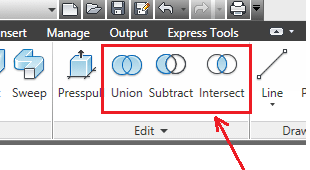The UNION command

The UNION command is simply the command that allows to unite 2 objects and make them be one.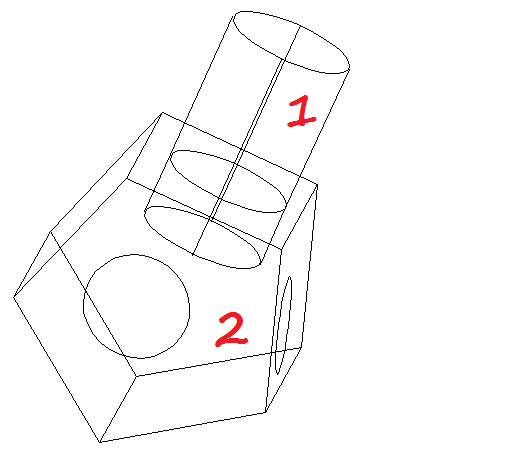Lets consider the figure above. The cylinder and the figure 2 are clearly two different objects. Uniting these two figures will result in a figure more or less identical to the original image above. But then you would not be able to move or change the other without affecting the whole.

To use the UNION command, you simply have to click on the UNION icon, select the two objects to join and press ENTER on your keyboard. The result of uniting the objects above will be the following.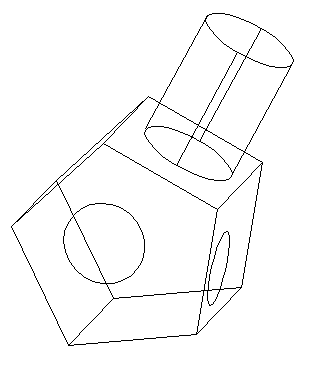Hard to notice the difference. The segment which was common to the both solids is gone, no more visible. Now the two solids are one object as a whole.

Most 3D object are association of many “sub-object”, and most time there is a need to draw every single part on their own and join all at the end to make the whole. This is where the UNION command is most needed.

The SUBTRACT command

The SUBTRACT command works a little like the UNION command. But in this case we subtract an object A from an object B. This command makes sense only when both object have an area which is common to both. Taking the figure above, where we have an object 1 and an object 2, the subtraction of 1 from 2 will give us the result below.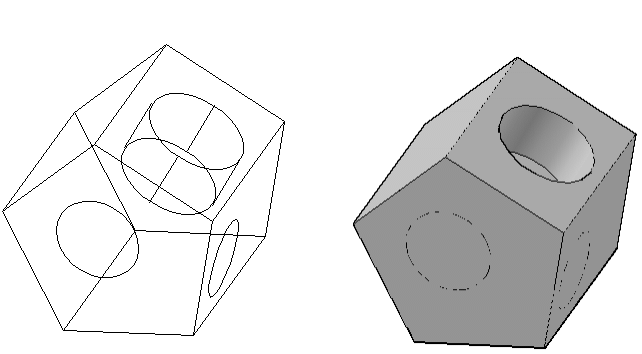You can notice there is a hole that appeared somewhere where the cylinder was. The region that was common to both object desapeared and no longer exist. The cylinder is gone too.

The SUBTRACT command can go both ways but each result is different. Subtracting 1 from 2 is different to subtracting 2 from 1. Try the opposite to see the difference (Subtracting 2 from 1)

To use the SUBTRACT command Click on the SUBTRACT icon, Select the first object and press ENTER, and Select the second object and press ENTER. Notice here the difference from the UNION command, you need to press ENTER between the selection of the two objects.

The INTERSECT command

The INTERSECT command is to be left with the area with is common to both solids. Using our example, if we use the INTERSECT command we will be left with the figure below.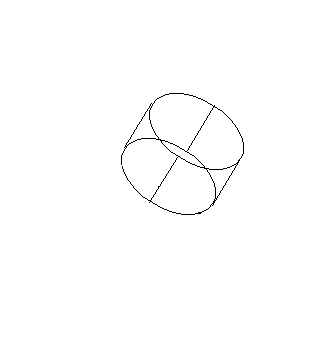Do not worry about mastering all command at once, you will understand them better with practice.